# Electronics and Communication Engineering - Electromagnetic Field Theory

31.
Gain of an antenna with a circular aperture of diameter 4 meter at frequency of 10 GHz, with directivity 60
60
100
30
90
Explanation: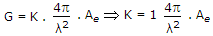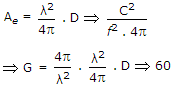32.
If the electric field intensity is given by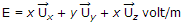, the potential difference between x(2, 0, 0) and y(1, 2, 3) is
+1 volt
-1 volt
+5 volt
+6 volt
Explanation: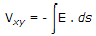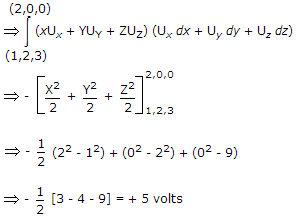33.
Medium 1 has the electrical permittivity ε1 = 1.5 ε0 farad/m and occupies the region to the left of x = 0 plane. Medium 2 has the electrical permittivity ε2 = 2.5 ε0 farad/m and the occupies the region to the right of x = 0 plane. If E1 in medium 1 is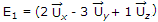volt/m, then E2 in medium 2 is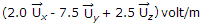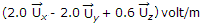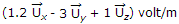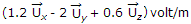Explanation: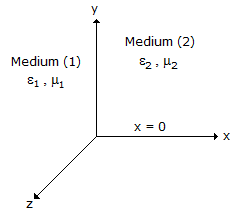According to boundary condition

hence, Ez1 = Ez2

Ey2 = Ey1

Et1 = Et2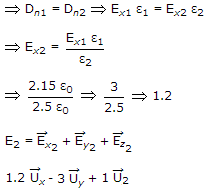34.
Polarization of a wave is
direction of electric field
direction of magnetic field at some fixed point in space
either (a) or (b)
none of the above
Explanation:

Direction of electric field is to be taken as reference.

35.
Calculate the critical frequency for the E layer if maximum ionic densities are 3 x 106
15.58 x 106
15.58 kHz
1.58 x 103
None of the above
fc = 9Nmax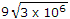15.58 x 103 Hz.